Home  |  Contact
 DESENMASCARANDO LAS FALSAS DOCTRINAS

 What’s NewMessage Board
Image Gallery
Files and Documents
Polls and Test
Member List
YHWH (DIOS PADRE) EL UNICO DIOS
JESUCRISTO NUESTRO MESIAS JUDIO
LOS DIEZ MANDAMIENTOS DE LA BIBLIA
MEJORE SU CARACTER Y SU VIDA
BIBLIAS/CONCORDANCIA/LIBROS
MAYOR ENEMIGO DEL HOMBRE ES UNO MISMO
¿LA TORA ES MACHISTA? -MENSAJE ESOTERICO Y EXOTERICO
¿ES INMORTAL EL ALMA?- FALACIA DE LA ENCARNACION Y REENCARNACION
EL ISLAM TIENE ORIGEN UNITARIO ADOPCIONISTA
ANTIGUO TESTAMENTO-ESTUDIO POR VERSICULOS
NUEVO TESTAMENTO-ESTUDIOS POR VERSICULOS
NUEVO TESTAMENTO II-ESTUDIOS POR VERSICULOS
NUEVO TESTAMENTO III-ESTUDIOS POR VERSICULOS
CRISTO NO TUVO PREEXISTENCIA
¿QUE ES EL ESPIRITU SANTO?

Tools

General: GIZE=SPEED OF LIGHT=JOHN 8:12=22/7 (SIMCHAT TORAH)=LEVITICUS 23:33
Choose another message boardPrevious subject  Next subjectReply Message 1 of 73 on the subject
 From: BARILOCHENSE6999  (Original message) Sent: 26/01/2021 17:34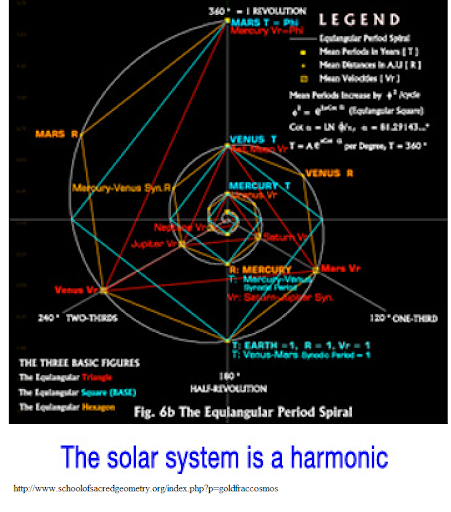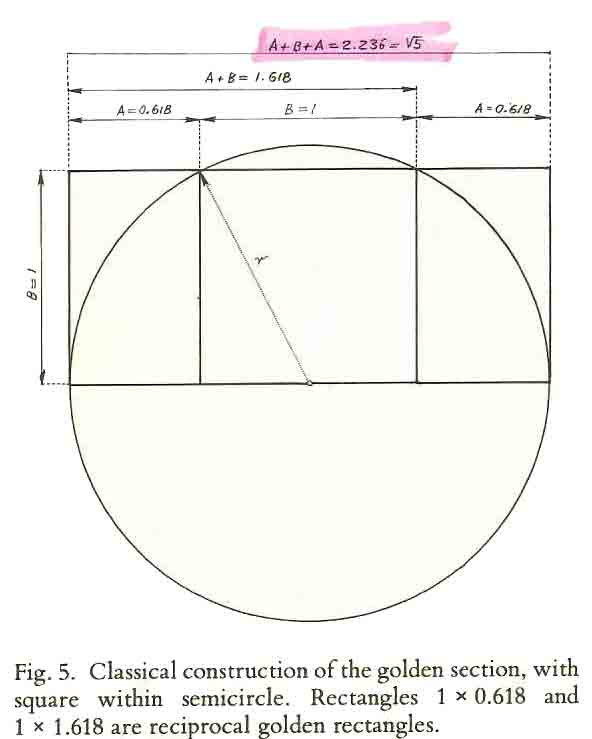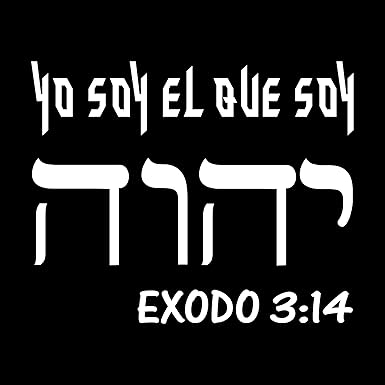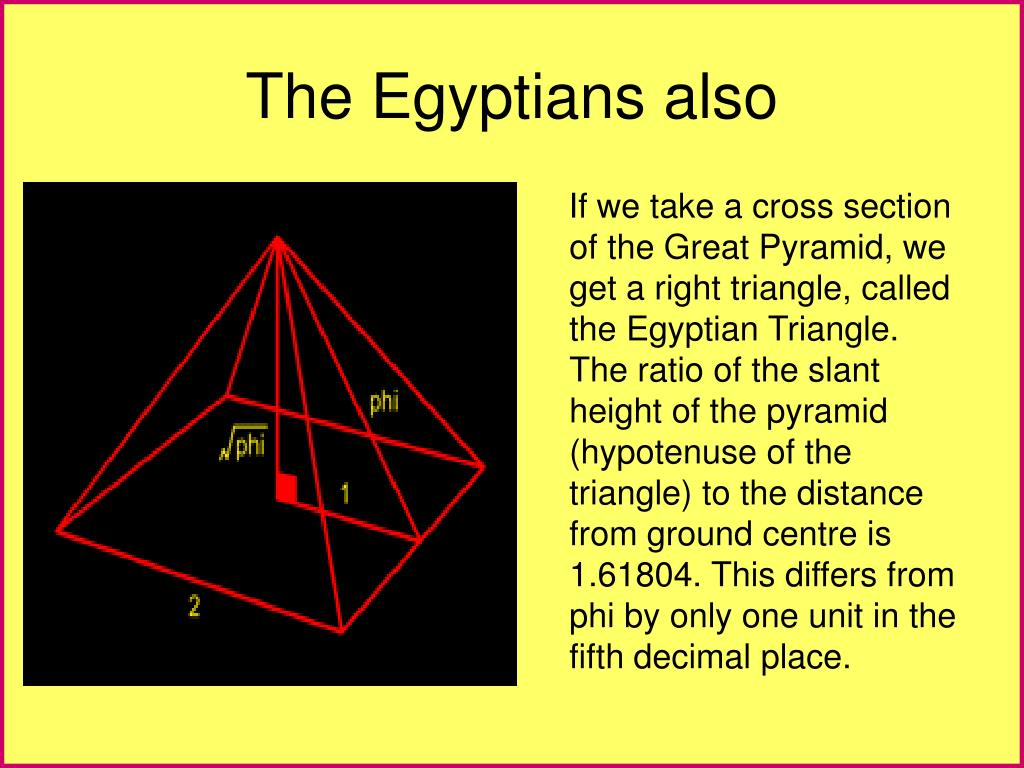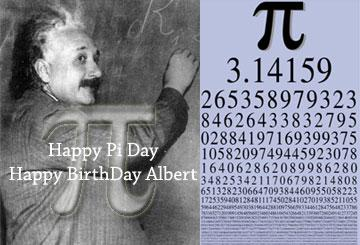22 de TISHRI=22/7=3.14

22/7=DIA DE MARIA MAGDALENA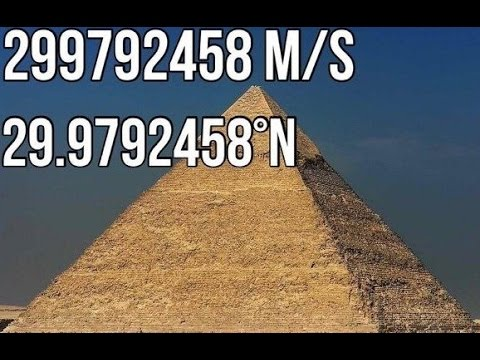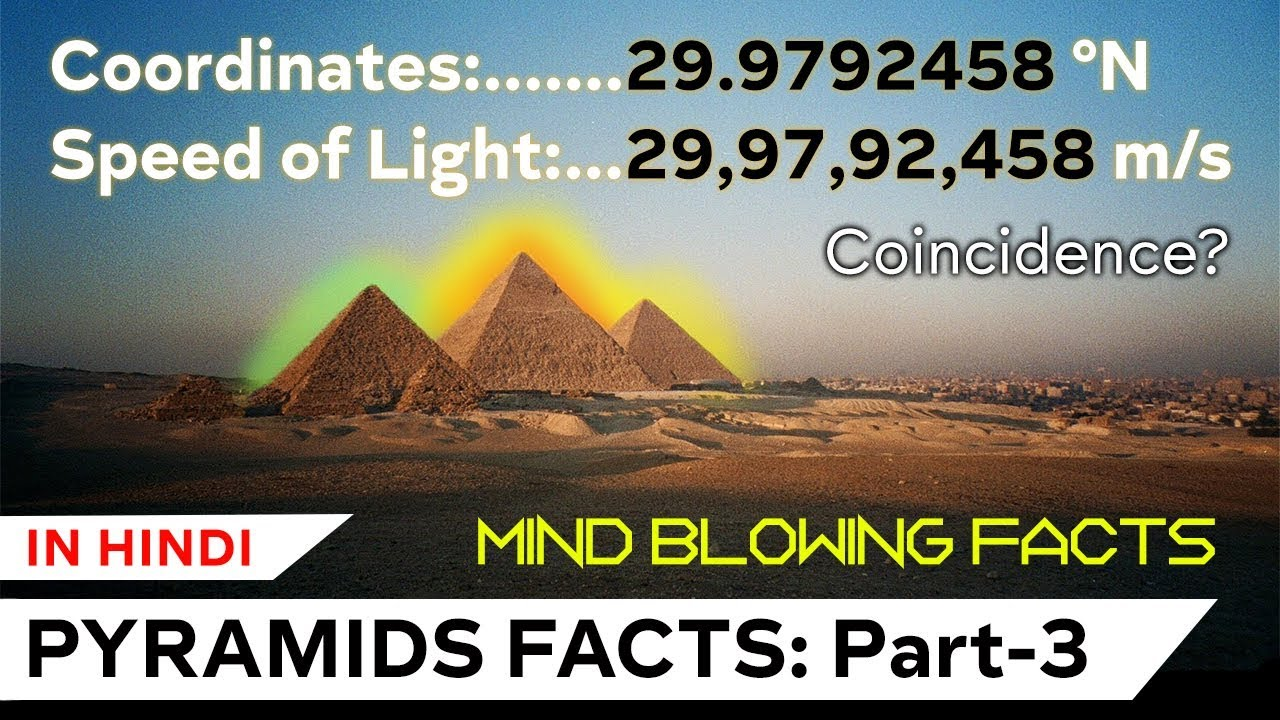John 8:12 G5457 saying, I am the light of the world: he that fo...

EN EL OCTAVO DIA DE LA FIESTA DE LOS TABERNACULOS, OSEA EL 22 DE TISHRI O 22/7 (LEVITICO 23:33)FirstPrevious  59 to 73 of 73  NextLastReply Message 59 of 73 on the subject
 From: BARILOCHENSE6999 Sent: 31/07/2021 16:59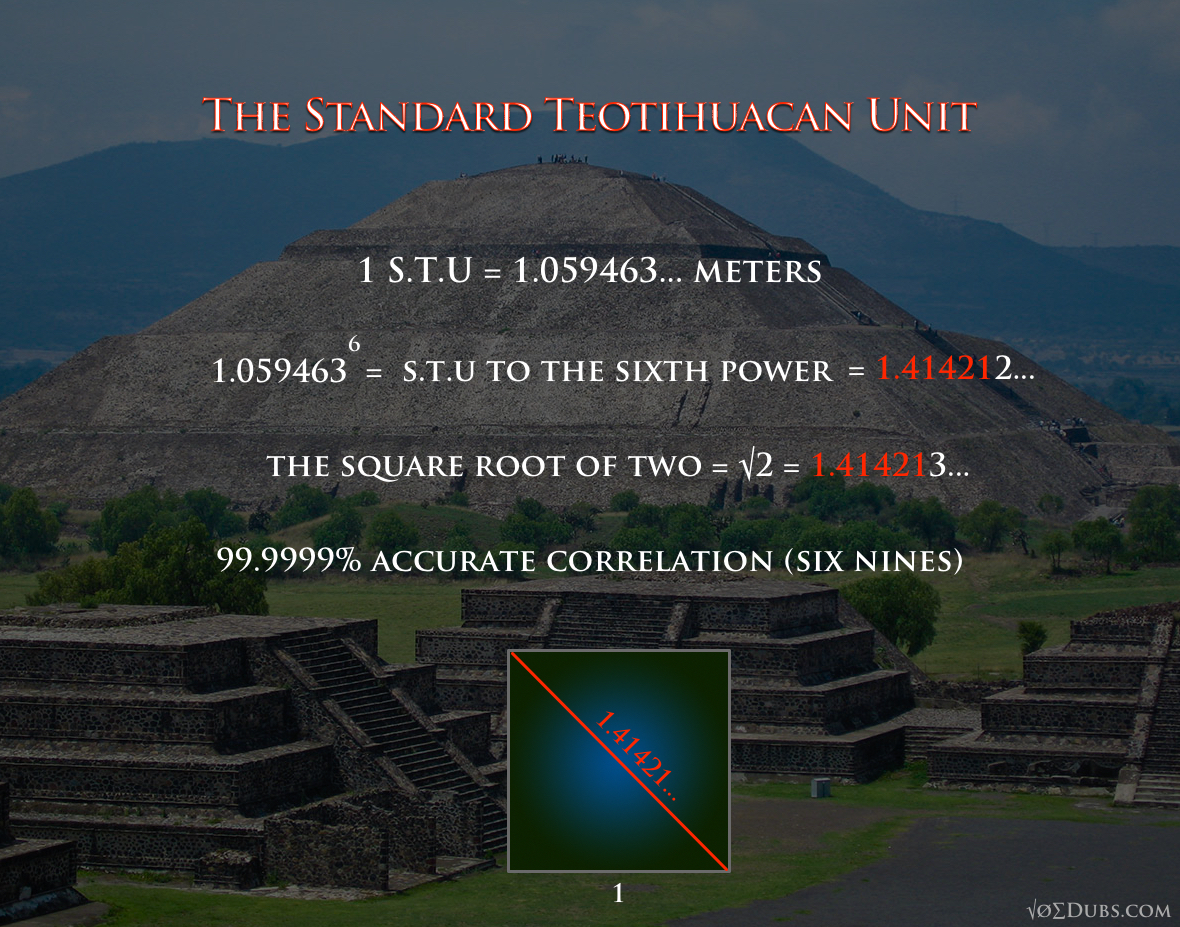NUMERO DE PLATA Numero de Plata=1.732=Raiz cuadrada 2; Numero de Platino=Raiz cuadrada 3=1.732

 Respuesta Mensaje 21 de 21 en el tema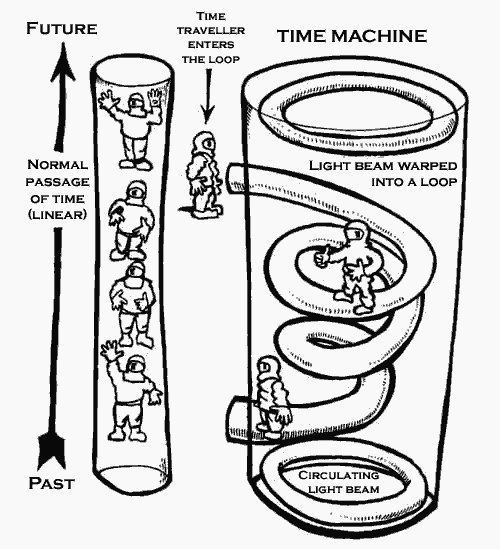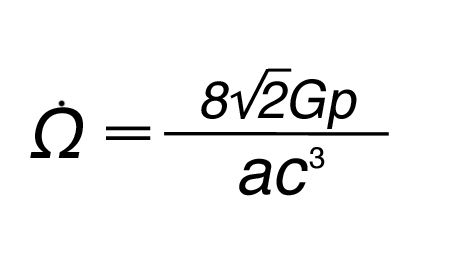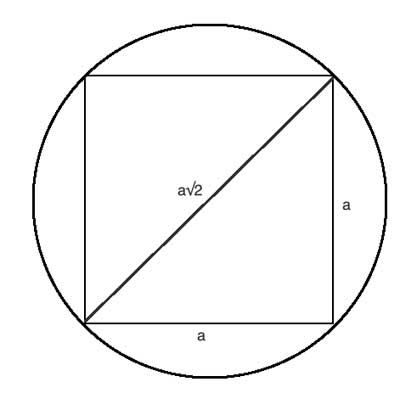Reply Message 60 of 73 on the subject
 From: BARILOCHENSE6999 Sent: 01/08/2021 00:51Reply Message 61 of 73 on the subject
 From: BARILOCHENSE6999 Sent: 01/08/2021 01:45## The Great Pyramid and the Speed of Light

The Great Pyramid encodes enormous amount of numerical coincidences ( pi, Phi, dimensions and movement of our planet, axial tilt, precession,  speed of light, and more…)
We can only wonder if the ancient architects were fully aware of these special numbers encoded in their design — or are these numbers simply the  result of  selecting 2 numbers (7 and 11) for proportions for the Great Pyramid???

 The design of the Great Pyramid is based on the ratio 11:7.  This ratio (equal 1.571) is perfect approximation of the “squaring the circle” principle.For the Great Pyramid, Base to Height Ratio 440/280 is exactly 11/7 Most “pyramidologists” appear to be  “chasing their tails” uncovering huge amount of “numerical coincidences” embedded in the Great Pyramid… It is simply unbelievable, however ALL of these numerical coincidences are result of selecting just 4 numbers for the pyramid design: 7, 11 (height to base ratio), 40 (the scale factor), and the 4th key number is the value of the measuring unit: Royal Cubit = 20.62 ” = 0.524 m.

This single, fundamental design principle: 11 : 7 Base to Height Ratio  generates ALL amazing mathematical properties of the Great Pyramid:

• the Golden Ratio Phi=1.618 (the Great Pyramid is a Golden Pyramid: length of the slope side (356) divided by half of the side (440/2 = 220) height is equal to 1.6181818… which is the Golden Ratio Phi
• squaring the circle ratio 1.571 (base/height = 44/28 = 1.571)
• pi=3.14159… (2 x base/height = 2 x 44/28 = 3.14286 which is very close approximation of “pi” = 3.14159…)
• Perimeter of the square base, 4×440=1760, is the same as circumference of the circle with radius = height: 2x ”pi” x height (2x 22/7 x 280=1760)
• The ratio of the perimeter to height of 1760/280 cubits equates to 2x pi
to an accuracy of better than 0.05%
• Side of the base (440) plus double height (2x 280=560) = 1,000
• Perimeter of the square base is equal 4×440=1760 RC = 0.5 nautical mile = 1/7,200th of the radius length of the earth
• the slop angle 51°.843
• The Pyramid exhibits in the design both pi and by Phi,  given the similarity
of 2/ sqrt(phi) (2 divided by the square root of Phi) with pi/2 :
• 11/ 7 equal 1.5714
• 2/ sqrt(89/55) equal 1.5722
• 2/ sqrt(Phi) equal 1.5723
• pi/ 2 equal 1.5708
• Royal Cubit = 0.5236 m,  pi – Phi2 = 0.5231
• and more…

### Does Great Pyramid encode “fractal” value of the speed of light?

The speed of light in a vacuum is 299, 792, 458 meters per second or 983,571,056.43045 feet per second or 186,282.397 miles per second.

Base of the Great Pyramid is a square with side B = 44o Royal Egyptian Cubits.  Let’s draw two circles: one inscribed and one superscribed on the square of the base of the Great Pyramid.• Circumference of superscribed circle: 2x pi x R
• Circumference of the inscribed circle: 2x pi x r

The difference of the circumference of both circles (lets call it C) is:

C = 2 x pi x (R – r) = 2 x pi x [ B/sqrt(2) - B/2 ] =
2x pi x B x [ 1/sqrt(2) - 1/2 ] = 1.301290285 x B

### The length of the Egyptian Royal Cubit

Based on The Pyramids and Temples of Gizeh” by W.M. Flinders Petrie. 1883.

The unit of measuring length used by the ancient architects in the construction of the Great Pyramid was the Royal Cubit.

Petrie estimated the value of the Royal Cubit using some key dimensions of the Great Pyramid:
By the base length of the Pyramid, if 440 cubits (section ’43): 20.611 ± .002

By the base of King’s Chamber, corrected for opening of joints:  20.632 ± .004 inches
By the Queen’s Chamber, if dimensions squared are in square cubits:  20.61   ± .020
By the antechamber: 20.58   ± .020
By the ascending and Queen’s Chamber passage lengths (section 149):  20.622 ± .002
By the gallery width:   20.605 ± .032

The Average value of the RC  (based on above numbers) is 20.61 inches.

It’s almost universally accepted that archaeologist Flinders Petrie’s determination of the royal cubit length at 20.632 inches, from his measurements of the King’s Chamber in the Great Pyramid of Giza, was the likely measure to survey the dimensions of that pyramid, 440 royal cubits per base side, but the experts stop there, not then letting you know that those 1,760 royal cubits which total the Great Pyramid’s base perimeter length, when multiplied by 20.632 inches, equals half a modern nautical mile, or 1/7,200th of the radius length of the earth, so there certainly is a connection.

 Reply Message 62 of 73 on the subject
 From: BARILOCHENSE6999 Sent: 02/08/2021 01:06

 Reply Message 63 of 73 on the subject
 From: BARILOCHENSE6999 Sent: 04/08/2021 01:26Reply Message 64 of 73 on the subject
 From: BARILOCHENSE6999 Sent: 08/08/2021 12:39

 Reply Message 65 of 73 on the subject
 From: BARILOCHENSE6999 Sent: 08/08/2021 22:37

 Reply Message 66 of 73 on the subject
 From: BARILOCHENSE6999 Sent: 30/08/2021 23:33
 (1) I begin the demonstration with a typical set of nested squares & circles. This diagram is very easy to construct with unmarked compass & straightedge, so I won’t bother with extraneous details. Although measurement is not used to construct these figures, it is obviously required for the proof. Therefore, I will treat the innermost Green Circle as a Unit Circle; (Radius = 1, Area = Pi). The outer Blue Circle is twice the size of the Green Circle; (Radius = √2, Area = 2Pi). Also, you will notice that I’ve labeled key intersecting points with the letters A-E, and made two line extensions; one in the center & one at the bottom. These will come into play in succeeding diagrams. http://www.circleissquared.com/

 Reply Message 67 of 73 on the subject
 From: BARILOCHENSE6999 Sent: 30/08/2021 23:40Reply Message 68 of 73 on the subject
 From: BARILOCHENSE6999 Sent: 01/09/2021 23:26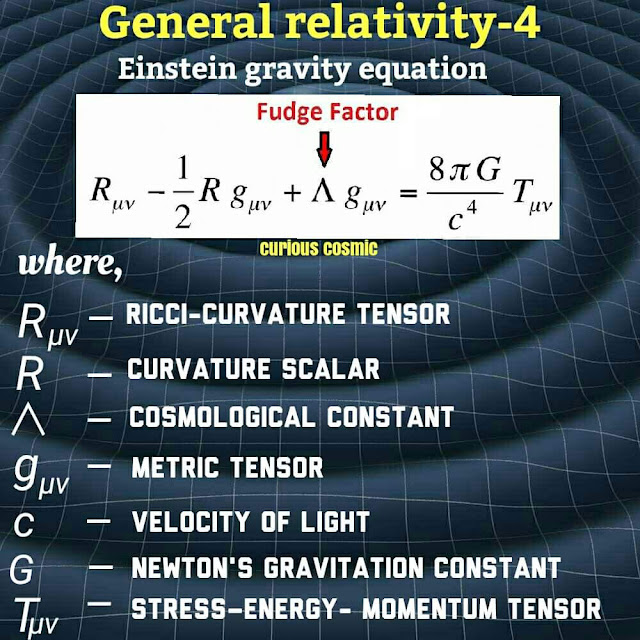Reply Message 69 of 73 on the subject
 From: BARILOCHENSE6999 Sent: 03/09/2021 20:30

 Reply Message 70 of 73 on the subject
 From: BARILOCHENSE6999 Sent: 07/09/2021 10:46

 Reply Message 71 of 73 on the subject
 From: BARILOCHENSE6999 Sent: 08/09/2021 00:06Reply Message 72 of 73 on the subject
 From: BARILOCHENSE6999 Sent: 16/09/2021 02:08

 Reply Message 73 of 73 on the subject
 From: BARILOCHENSE6999 Sent: 16/09/2021 23:07
 The amount of days in a solar year plus the proportions for the Equatorial circumference of the Earth and the proportions of the Great Pyramid of Giza according to Golden Pi = 4/√φ = 3.144605511029693144: The Great Pyramid of Giza is a geodetic model of Planet Earth. The measurements mentioned below are the ideal measurements. A meter is equal to 100 centimeters 1 Solon cubit = 40 times √φ = 50.88078598056276 centimeters. If 1 Solon cubit is divided into 20 equal units of measure then 20 inches can be derived because 1 Solon cubit is equal to 20 inches. 1 Saylen cubit = 50 times √φ = 63.60098247570345 centimeters. If 1 Saylen cubit is divided into 25 equal units of measure then 25 inches can be derived because 1 Saylen cubit is equal to 25 inches. 1 inch = 2 times √φ = 2.544039299028138 centimeters. 1 foot = 12 inches. 1 foot = 24 times √φ = 30.528471588337656 centimeters. If the shortest edge length of a Kepler right triangle is equal to 1 then the hypotenuse of the Kepelr right triangle is equal to The Golden ratio = (√(5) plus 1)/2 = φ = 1.618033988749895 according to the Pythagorean theorem. The Golden ratio in Trigonometry = (cosine (36 degrees) times 2) = 1.618033988749895. If the shortest edge length of a Kepler right triangle is equal to 1 then second longest edge length of the Kepelr right triangle is the square root of the Golden ratio = √φ = 1.272019649514069 according to the Pythagorean theorem. The width for the square base of the Great Pyramid of Giza is equal to 756 feet. • If the shortest edge length of a Kepler right triangle is equal to 1 foot and then the second edge length of the Kepler right triangle is multiplied 378 equal times the result will be the height of the Great Pyramid of Giza = 378 times √φ = 480.823427516318082 feet according to Golden Pi = 4/√φ = 3.144605511029693144. • If the shortest edge length of a Kepler right triangle is equal to 1 foot and then the hypotenuse of the Kepler right triangle is multiplied 378 equal times the result will be the slant height of the Great Pyramid of Giza = 378 times φ = 611.61684774746031 feet according to Golden Pi = 4/√φ = 3.144605511029693144. • If the shorter edge length of a Golden ratio = (√(5) plus 1)/2 = φ = 1.618033988749895 rectangle is equal to 1 foot and then the diagonal of the Golden ratio = (√(5) plus 1)/2 = φ = 1.618033988749895 rectangle is multiplied 378 equal times the result will be the edge height of the Great Pyramid of Giza = 378 times = Cosine (18) degrees times 2 = 1.902113032590307 = 718.998726319136046 feet according to Golden Pi = 4/√φ = 3.144605511029693144.Cosine (18) degrees times 2 = 1.902113032590307. Cosine (18) degrees times 2 = 1.902113032590307 squared = φ plus 2 = 3.618033988749895. • If the shortest edge length of a Kepler right triangle is equal to 1 foot = 24 times √φ = 30.528471588337656 centimeters then the second longest edge length of that Kepler right triangle is equal to 38.832815729997479 centimeters.24 times √φ = 30.528471588337656 centimeters times the square root of the Golden ratio = √φ = 1.272019649514069 = 38.832815729997479 centimeters. 1 foot = 24 times √φ = 30.528471588337656 centimeters times the square root of the Golden ratio = √φ = 1.272019649514069 = 38.832815729997479 centimeters times 378 = the height of the Great Pyramid of Giza of Giza = 14678.804345939047062 centimeters. The height of the Great Pyramid of Giza = 14678.804345939047062 centimeters divided by 24 times √φ = 30.528471588337656 centimeters = the height of the Great Pyramid of Giza = 480.823427516318088 feet. 378 times √φ = 1.272019649514069 = 480.823427516318088. • If the shortest edge length of a Kepler right triangle is equal to 1 foot = 24 times √φ = 30.528471588337656 centimeters then the hypotenuse of that Kepler right triangle is equal to 49.39610465451582 centimeters. 24 times √φ = 30.528471588337656 centimeters times the Golden ratio = (√(5) plus 1)/2 = φ = 1.618033988749895 = 49.39610465451582 centimeters. 1 foot = 24 times √φ = 30.528471588337656 centimeters times the Golden ratio = (√(5) plus 1)/2 = φ = 1.618033988749895 378 = 49.39610465451582 centimeters times 378 = slant the height of the Great Pyramid of Giza = 18671.727559406979971centimeters. The Slant height of the Great Pyramid = 18671.727559406979971 centimeters divided by 24 times √φ = 30.528471588337656 centimeters = the slant height of the Great Pyramid of Giza = 611.61684774746031 feet. 378 times √φ = 1.272019649514069 = 611.61684774746031. • If the shortest edge length of a Golden ratio = (√(5) plus 1)/2 = φ = 1.618033988749895 rectangle is equal to 1 foot = 24 times √φ = 30.528471588337656 centimeters then the length of the diagonal of that Golden ratio = (√(5) plus 1)/2 = φ = 1.618033988749895 rectangle is equal to 58.068603673239965 centimeters. 24 times √φ = 30.528471588337656 centimeters times Cosine (18) degrees times 2 = 1.902113032590307 = 58.068603673239965 centimeters. Cosine (18) degrees times 2 = 1.902113032590307 squared = φ plus 2 = 3.618033988749895. 1 foot = 24 times √φ = 30.528471588337656 centimeters times = Cosine (18) degrees times 2 = 1.902113032590307 = 58.068603673239965 centimeters times 378 = the edge height of the Great Pyramid of Giza of Giza = 21949.932188484706835 centimeters. The edge height of the Great Pyramid = 21949.932188484706835 centimeters divided by 24 times √φ = 30.528471588337656 centimeters = the edge height of the Great Pyramid of Giza = 718.998726319136046 feet. 378 times Cosine (18) degrees times 2 = 1.902113032590307 = 718.998726319136046. Cosine (18) degrees times 2 = 1.902113032590307. Cosine (18) degrees times 2 = 1.902113032590307 squared = φ plus 2 = 3.618033988749895. A Kepler right triangle can be created from the construction of a Golden ratio = (√(5) plus 1)/2 = φ = 1.618033988749895 rectangle by using Compass and straight edge and obviously a marker for the drawing surface. The amount of days in a Solar year = 4/√φ times 7920 times 5280/(10 ^ 3 times 360) = 365.277376161209156. The amount of days in a Solar year = 4/√φ = 3.144605511029693144 times 7920 times 5280/(10 ^ 3 times 360) = 365.277376161209156. The equatorial circumference of planet Earth = 10 ^ 3 times 360 times 365.277376161209156 = 131499855.41803529616 feet. The equatorial circumference of planet Earth = 4/√φ = 3.144605511029693144 times 7920 = 24905.275647355169727 statute miles. 131499855.41803529616 feet divided by 86400 = half the perimeter of the socle of the Great Pyramid of Giza = 1521.989067338371483 feet. Half the perimeter of the socle of the Great Pyramid of Giza times 86400 is also equal to the equatorial circumference of planet Earth = 131499855.41803529616 feet. 484 divided by √φ times 2 = the width of the socle of the Great Pyramid of Giza = 760.99453366918572 feet. Half the width of the socle of the Great Pyramid of Giza = 380.49726683459286 feet times √φ = 484 feet. 484/√φ times 2 times 2 times 86400 = 131499855.41803529616 feet. 131499855.41803529616 feet divided by 5280 = The perimeter of the socle of the Great Pyramid of Giza = 484/√φ times 8 = 3043.978134676742966 feet. 484 feet /√φ times 8 = 3043.978134676742966 feet times 12 = 24905.275647355169727 statute miles. 24905.275647355169727 statute miles divided by 7920 statute miles = Golden Pi = 4/√φ = 3.144605511029693144. There are 929.28 meters in the square perimeter of the socle of the Great Pyramid of Giza according to Golden Pi = 4/√φ = 3.144605511029693144. 484/√φ times 8 = 3043.978134676742966 times 12 = 36527.737616120915592 divided by 100 = the exact amount of days in a solar year = 365.277376161209156. The equatorial diameter of our planet Earth = 41817600 feet. 41817600 feet divided 86400 = the height of the Great Pyramid of Giza = 378 times √φ = 480.823427516318082 feet plus the height of the socle of the Great Pyramid of Giza = 3.176572483681918 feet. 10 ^ 3 times 360 times 484/(√φ) times 8 times 12/(100) = 131499855.41803529616 feet. The height of the Great Pyramid if Giza is 378 times √φ = 480.823427516318082 feet. The width of the square base of the Great Pyramid of Giza is 756 feet. The perimeter of the square base of the Great Pyramid of Giza = 3024 feet. 9 factorial = 362880. At 10 degrees latitude the length of a degree is 9 factorial =362880 feet. The amount of inches in the perimeter of the square of the Great Pyramid of Giza = 36288. 36288 times 10 = 362880. The width for the square base of the Great Pyramid of Giza = 756 feet. The perimeter of the square base of the Great Pyramid of Giza = 3024 feet. There are 36288 inches in the perimeter of the square base of the Great Pyramid of Giza. 3024 times 12 = 36288. 756 times 4 times 12 = 36288. (9!)/10 = 36288. The equatorial circumference of planet Earth: https://joedubs.com/four-earthly-elements/equatorial-circumference-of-earth/ Kepler right triangle diagram with squares upon the edges of the Kepler right triangle: https://drive.google.com/file/d/1iBtXYy06yv9UWtGP5mwMXyt80vaysFvR/view?usp=sharing Kepler right triangle construction method: https://drive.google.com/file/d/15DNXB_xNP2f2jCroC0FyNUyBYGhVAA2J/view?usp=sharing PYTHAGOREAN THEOREM: https://en.wikipedia.org/wiki/Pythagorean_theorem Golden ratio: https://en.wikipedia.org/wiki/Golden_ratio The history of the meter: The history of the meter: https://www.factinate.com/editorial/meter-history/ The meter: https://en.wikipedia.org/wiki/Metre The meter is based now on the speed of light: https://www.youtube.com/watch?v=vgqUyFaUDcIFirstPrevious  59 a 73 de 73  NextLastPrevious subject  Next subject